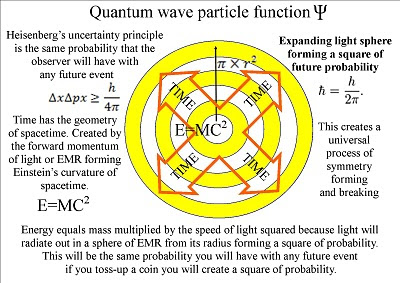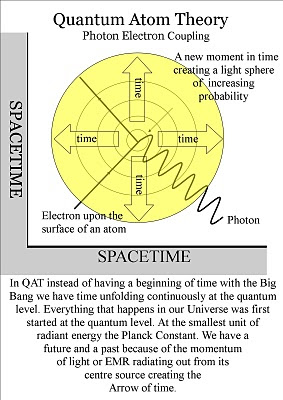Sunday, 11 July 2010

A concept of Time an artist theory

A concept of Time an artist theory
~

In this theory Time and the geometry of Spacetime is continuously being formed by the spontaneous absorptions and emission of light or EMR, at the quantum level of the atoms.Electrons in atoms can change energy levels by emitting or absorbing a photon of light whose energy must be exactly equal to the energy difference between the two levels. The frequency of light emitted is a function of how far away in energy the two states of the system were, the energy difference between the two states equals the energy carried off by the new photon. This new photon will therefore have an energy level of the Golden ratio compared to the previous energy levels.The mathematics of the golden ratio and of the Fibonacci sequence 0, 1, 1, 2, 3, 5, 8, 13, 21, 34, 55, 89, 144, 233, 377, 610, 987, … are intimately interconnected.This gives us a geometrical therefore mathematical base for the evolution of life explaining why Nature never produces straight lines but an endless array of curves and spirals.We therefore have a universal process of symmetry forming and breaking. Formed by the spherical shape of the quantum wave particle function in three dimensional space forming Einstein’s curvature of spacetime.

This can also be seen in the size of the fine structure constant 137… that cannot be explained by modern physics.In this theory the fine structure constant results from dividing a sphere into parallel bands of equal area and placing one node in each band at longitudes spaced by a golden section of the circle, i.e. 360°/φ ≅ 222.5°. We then subtract this from 360. The result will always be 137.5 that why it's a constant.The fine structure constant 137… is the symmetry and geometry of spacetime formed by an interaction between electromagnetic radiation (light) and the spherical electron cloud around an atom.

This is why we have 4pi in the equation representing the shape of the wave function in three dimensional spacetime.We also have pi in the equation for Heisenberg Uncertainty Principle represents the same uncertainty the observer will have with any future event.
~

A link to Quantum Atom Theory on YouTube.

~